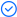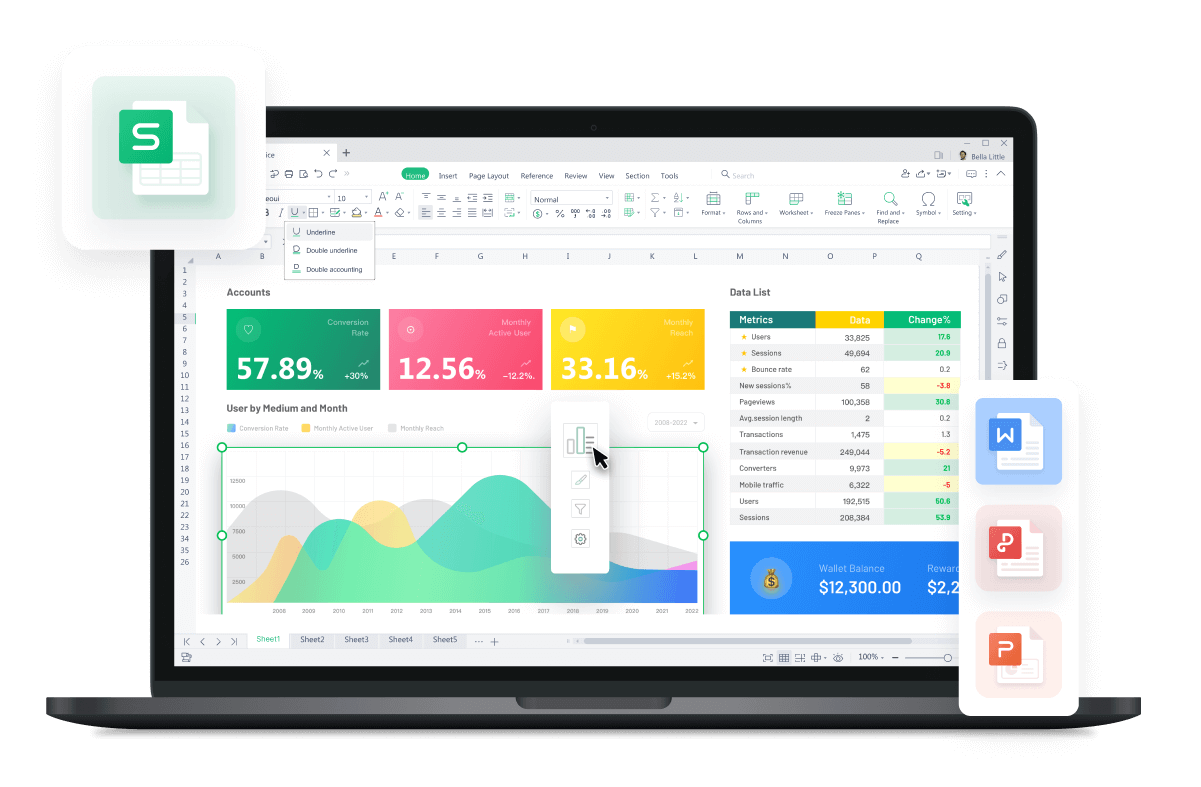WPS Office

Free All-in-One Office Suite with PDF EditorRead, edit, and convert PDFs with the powerful PDF toolkit.Microsoft-like interface, easy to use.

Windows • MacOS • Linux • iOS • Android# How to use monthly payment formula in excel with examples

July 22, 2022
3.3K Views

A loan payment is a monthly you have to make each month. This includes various factors such as interest, principle and any fees that are associated with the loan. In order to calculate the most accurate monthly payment on a loan, you should know not only how to calculate interest but also what drivers may affect your monthly payment. For example, when paying on a loan, one of the largest items affecting your monthly payment is the interest rate.

Calculating monthly loan payments in Excel can be very tricky at first. Many people find themselves confused by the process of calculating a personal loan payment. There are various variables that need to be considered, and how much you owe depends on each individual scenario. This guide will help you determine monthly payments like house mortgage, car loan, and student loan payments using monthly payment formula in excel.

How to use monthly payment formula in excel to calculate mortgage monthly payments

You can use PMT formula to calculate monthly loan payments in excel. But to use PMT formula and get correct results, you need to know interest rate, duration of loan, and amount borrowed. For the sake of using PMT formula, we are going to use a table containing all the necessary information. You can use the following steps to calculate monthly payments in excel using the PMT formula.

This method works best with versions 2016/2016/mac/online.

1. First of all, select the cell where you want to calculate the monthly payments.

1. After you have selected the cell, write the PMT formula in the formula bar as it is written here.

1. After you have written the PMT formula, hit enter and the monthly payment would appear in the cell.

Please note that the monthly payment in negative indicates this amount is being spent monthly.

How to use monthly payment formula in excel to calculate car payments

Using Excel to calculate a monthly automobile payment is similar to calculating a monthly mortgage payment. To begin, you'll need the interest rate, loan term, and loan amount.

You can use the following steps to calculate monthly car payments in excel using the PMT formula.

This method works best with versions 2016/2016/mac/online.

1. First of all, select the cell where you want to calculate the monthly payments.

1. After you have selected the cell, write the PMT formula in the formula bar as it is written here.

1. After you have written the PMT formula, hit enter and the monthly payment would appear in the cell.

How to use monthly payment formula in excel to calculate student loan payments

1. First of all, select the cell where you want to calculate the monthly payments.

1. After you have selected the cell, write the PMT formula in the formula bar as it is written here.

1. After you have written the PMT formula, hit enter and the monthly payment would appear in the cell